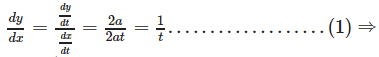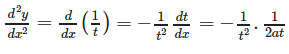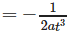# Test: Continuity And Differentiability (CBSE Level) - 1

## 25 Questions MCQ Test Mathematics (Maths) Class 12 | Test: Continuity And Differentiability (CBSE Level) - 1

Description
This mock test of Test: Continuity And Differentiability (CBSE Level) - 1 for JEE helps you for every JEE entrance exam. This contains 25 Multiple Choice Questions for JEE Test: Continuity And Differentiability (CBSE Level) - 1 (mcq) to study with solutions a complete question bank. The solved questions answers in this Test: Continuity And Differentiability (CBSE Level) - 1 quiz give you a good mix of easy questions and tough questions. JEE students definitely take this Test: Continuity And Differentiability (CBSE Level) - 1 exercise for a better result in the exam. You can find other Test: Continuity And Differentiability (CBSE Level) - 1 extra questions, long questions & short questions for JEE on EduRev as well by searching above.
QUESTION: 1

###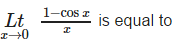Solution: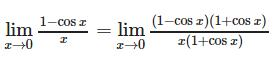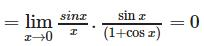QUESTION: 2

###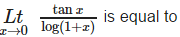Solution: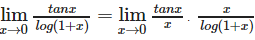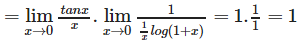QUESTION: 3

### Let f(x) = x – [x], then f ‘ (x) = 1 for

Solution:

f(x) = x -[x] is derivable at all x ∈ R – I , and f ‘(x) = 1 for all x ∈ R – I .

QUESTION: 4

f (x) = max {x, x3},then the number of points where f (x) is not differentiable, are

Solution:

f(x)=m{x,x3}
= x;x<−1 and
= x3;−1≤x≤0
⇒ f(x)=x;0≤x≤1 and
= x3;x≥1
∴ f(x)=1;x<−1
∴ f′(x)=3x2;− 1≤x≤0 and =1
0<x<1

QUESTION: 5

If f(x) = tan-1x and g(x) =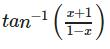, then

Solution: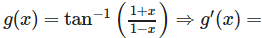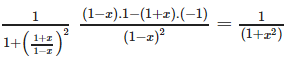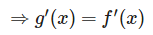QUESTION: 6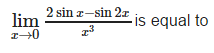Solution: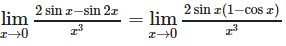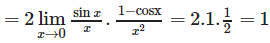QUESTION: 7

The function f (x) = 1 + | sin x l is

Solution:

f(x) = 1+|sinx| is not derivable at those x for which sinx = 0, however, 1+|sinx| is continuous everywhere (being the sum of two continuous functions)

QUESTION: 8

Let f (x + y) = f(x) + f(y) ∀ x, y ∈ R. Suppose that f (6) = 5 and f ‘ (0) = 1, then f ‘ (6) is equal to

Solution: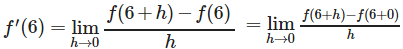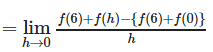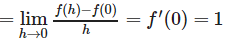QUESTION: 9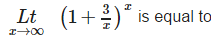Solution: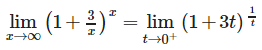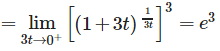QUESTION: 10

Derivative of log | x | w.r.t. | x | is

Solution:

d/dx(log|x|)
= 1/|x|

QUESTION: 11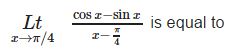Solution: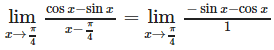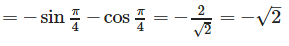QUESTION: 12

The function, f (x) = (x – a) sin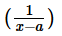for x ≠ a and f (a) = 0 is

Solution: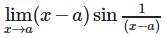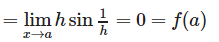QUESTION: 13

If x sin (a + y) = sin y, then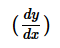is equal to

Solution:

x sin(a+y) = sin y
⇒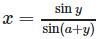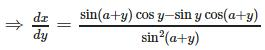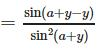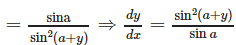QUESTION: 14Solution: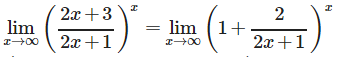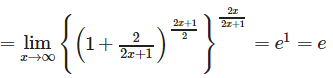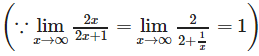QUESTION: 15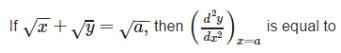Solution: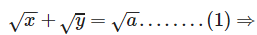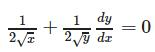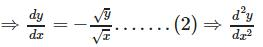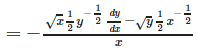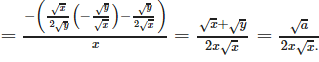QUESTION: 16

If [x] stands for the integral part of x, then

Solution: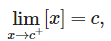If c is an integer , then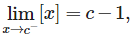Does not exist.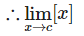QUESTION: 17

Let f (x) = [x], then f (x) is

Solution:

f(x) = [x] is derivable at all x except at integral points i.e. on R – I .

QUESTION: 18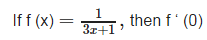Solution: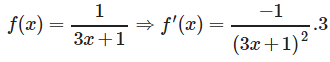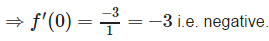QUESTION: 19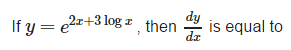Solution: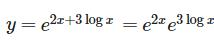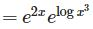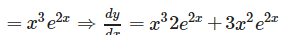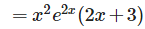QUESTION: 20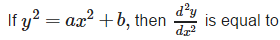Solution: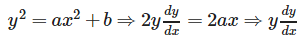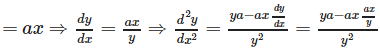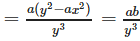QUESTION: 21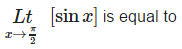Solution: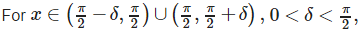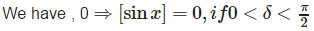QUESTION: 22

The function f (x) = [x] is

Solution:

The function f (x) = [x] isdiscontinuous only for all integral values of x.

QUESTION: 23

Let f be a function satisfying f(x + y) = f(x) + f(y) for all x, y ∈ R, then f ‘ (x) =

Solution: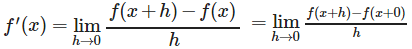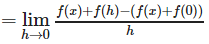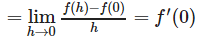QUESTION: 24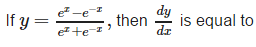Solution: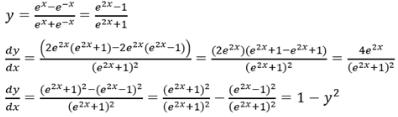QUESTION: 25

If x = at2, y = 2at, then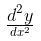is equal to

Solution: